# Cauchy characteristic problem

(diff) ← Older revision | Latest revision (diff) | Newer revision → (diff)

The problem of finding a solution of a partial differential equation (or a system of partial differential equations) which assumes prescribed values on a characteristic manifold.

There is a large class of equations of hyperbolic and parabolic types for which a non-closed-dimensional surface, oriented in a certain way, may serve as the initial surface in the spaceof independent variables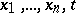. For example, ifis a space-like surface then the Cauchy problem (with initial data on) is always well-posed. In a characteristic Cauchy problem the initial surface is always a characteristic manifold (or a well-defined part thereof). In this case the Cauchy problem may have no solution at all; and if it has a solution it need not be unique.

For example, the characteristic Cauchy problem for the equation (,)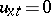with data on the characteristic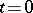: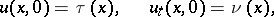is not well-posed. If the characteristic Cauchy problem has a solution, then the equation and the second initial condition imply a necessary condition for its solvability: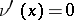, i.e. the characteristic Cauchy problem may be solvable only if. In that case, if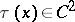,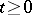, a solution indeed exists and is given bywhere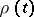is any function of class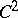,, satisfying the conditions.

A necessary condition for the existence of a solution to a characteristic Cauchy problem for a linear system of hyperbolic equations is that the rank of the augmented matrix of the system equals the rank of the singular matrix along the characteristic surface.

There is a wide class of hyperbolic equations and systems for which one may take a characteristic surface as an initial surface. For example, for the equation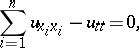(1)

when the characteristic surfaceis the cone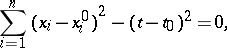(2)

the characteristic Cauchy problem may be phrased as follows: Find a solution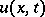of equation (1) which is regular within the cone (2) and takes prescribed values on the cone (2).

In the case of a space-like variable (,), the cone (2) is a pair of straight lines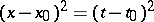passing through the point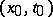. These straight lines divide the planeof the variables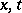into four angles. Let the domainbe one of these angles. Then the characteristic problem is customarily known as the Goursat problem: Determine a solutionof the equation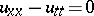which is regular inand satisfies the conditions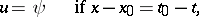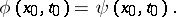If the characteristic surfaceis at the same time a surface of degenerate type or order, the characteristic Cauchy problem may prove to be well-posed.

For the equation(3)

which is hyperbolic for, the curve of degeneracy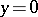is a characteristic. If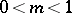, the Cauchy problem(4)

for equation (3) is well-posed, but if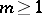it becomes ill-posed. In that case it is natural to investigate the problem either with modified initial data: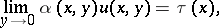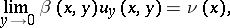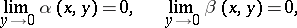or with incomplete initial data, i.e. dropping one of the conditions (4).

How to Cite This Entry:
Cauchy characteristic problem. Encyclopedia of Mathematics. URL: http://encyclopediaofmath.org/index.php?title=Cauchy_characteristic_problem&oldid=13736
This article was adapted from an original article by V.A. Eleev (originator), which appeared in Encyclopedia of Mathematics - ISBN 1402006098. See original article